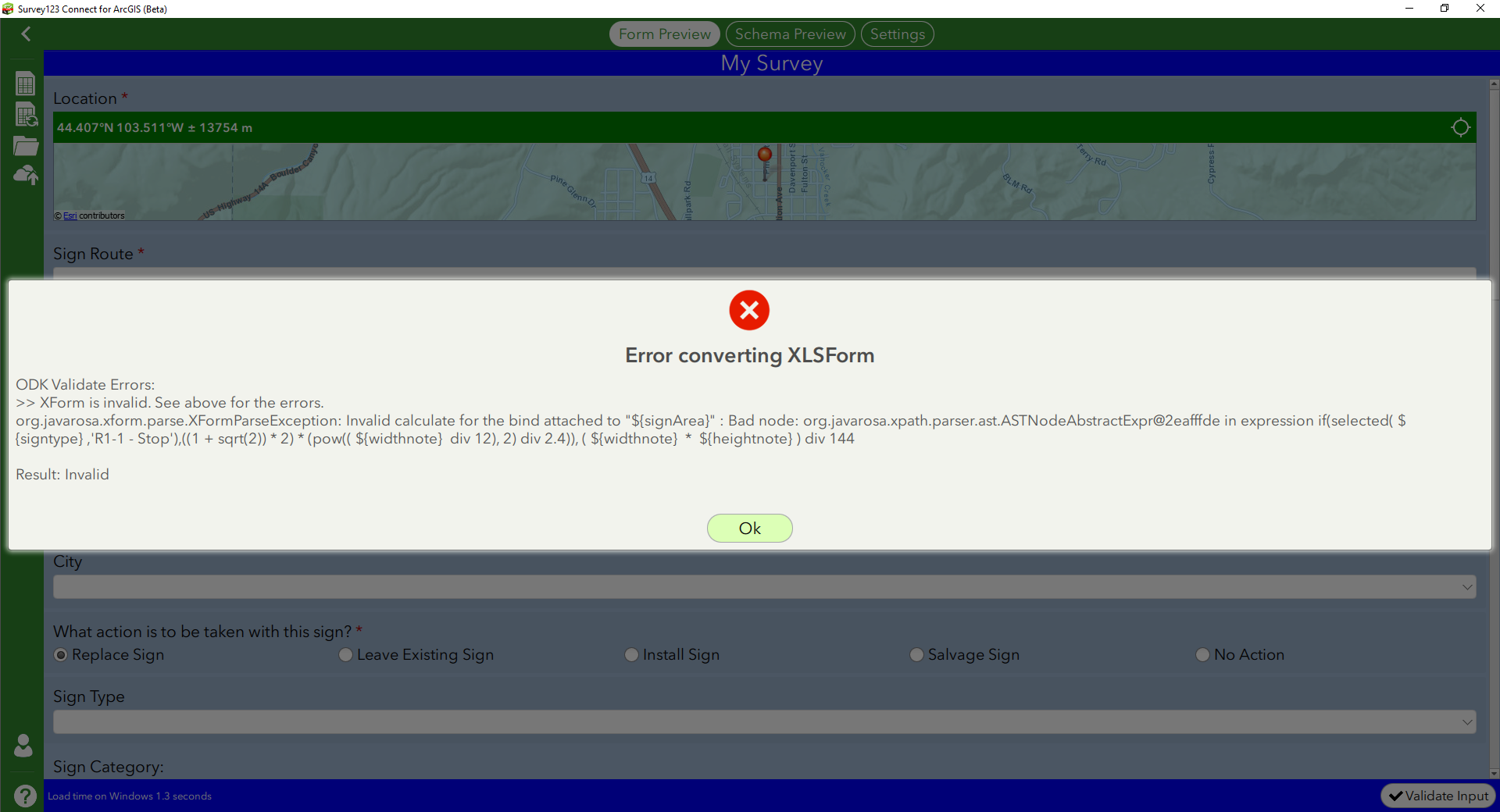# How can I calculate area when I have multiple equations for many feature types?

921
27
03-11-2019 02:51 PM
Highlighted
New Contributor III

Hi All,

I have another formula to create, but I am new to Survey123 and am not sure how to get what I need on the survey.  The project I am working on is sign inventory and the question I am having trouble with is with the area of each sign (200 different sign types).  I will have the width and height of each of the sign, but the signs, being all different shapes, have different area formulas.

These are all the different formulas:

(Width * Height)/144 (The majority of the signs have this equation)

(SQUARE(Height/12)) * .76

((1 + (SQRT(2))) * 2) * (SQUARE((Width / 12)/2.4))

(Height * Height /333)

(Width * Width * .0052083)

(Width)/12

(Width * Height)/182.5

(Height * Height * .0042918)

I have this for an equation (\${SignWidth}*(\${SignHeight})div144) for the majority of the signs, but the more advanced equations I am clueless on.  If you know how to write out some of these, that would be very helpful.  I am not even sure which survey column I should be writing these in either (calculation, relevent, etc.?)

I think I am in need of a very intense, nested if statement, but maybe it needs to be broken up in some way. I've included what my data looks like for reference.  Please help!

Tags (5)
27 Replies
Highlighted
byEsri Regular Contributor

Hi Kaitlyn

Your calculation for the majority of the signs is good, however you could drop the brackets around height, e.g. (\${width} * \${height}) div 144.

You should be able to specify a different equation for each sign type with a nested if statement in the calculation column. Please see this handy information on using formulas in calculations, which lists the functions and operators you can use (including if statements): Formulas—Survey123 for ArcGIS | ArcGIS

For example:

• (SQUARE(Height/12)) * .76 would be something like: pow((\${height} div 12), 2) * 0.76
• ((1 + (SQRT(2))) * 2) * (SQUARE((Width / 12)/2.4)) would look something like: ((1 + sqrt(2)) * 2) * (pow((\${width} div 12), 2) div 2.4)

Hope this helps.

Best regards,

Jim

Highlighted
New Contributor III

Would a nested if statement with two of your equations look something like this?

if(selected(\${signtype},'R1-1 - Stop'),((1 + sqrt(2)) * 2) * (pow((\${width} div 12), 2) div 2.4)), (\${width} * \${height}) div 144

This equation is not working for me.  I have never done any calculation this intense and have a lot of questions.

Highlighted
Frequent Contributor II

Missing the last )

if(selected(\${signtype},'R1-1 - Stop'),((1 + sqrt(2)) * 2) * (pow((\${width} div 12), 2) div 2.4)), (\${width} * \${height}) div 144)

Highlighted
New Contributor III

Here is what I get without that last 😞Here is the error with the extra 😞Highlighted
Frequent Contributor II

Opps see an extra  try

if(selected(\${signtype},'R1-1 - Stop'),((1 + sqrt(2)) * 2) * (pow((\${width} div 12), 2) div 2.4), (\${width} * \${height}) div 144)

Highlighted
New Contributor III

Thanks Doug!  That worked!  How many of questions am I able to add into a nested if statement?

These are all the 8 formulas I have:

(Width * Height)/144 (The majority of the signs have this equation)

(SQUARE(Height/12)) * .76

((1 + (SQRT(2))) * 2) * (SQUARE((Width / 12)/2.4))

(Height * Height /333)

(Width * Width * .0052083)

(Width)/12

(Width * Height)/182.5

(Height * Height * .0042918)

What if I have multiple sign types for each equation (can I go by category in the same equation)?  Should I put the equation with the most signs at the end of the equation?

Highlighted
Frequent Contributor II

As many as your brain can keep straight I think.

You could also maybe do one if per field then another field that just takes the value that is not 0.

So'

If (case 1, value, 0)

If (case 2, value, 0)

Then

Max(case 1, case 2)

Highlighted
New Contributor III

I'm not sure I know what you mean.  Do you mean I should expand my survey by adding more notes with subsequent signArea labels?

Like this?

note signArea Sign Area: if(selected(\${signtype},'R1-1 - Stop'),((1 + sqrt(2)) * 2) * (pow((\${widthnote} div 12), 2) div 2.4), 0)

note signArea Sign Area: if(selected(\${signtype},'M1-7 - NFS ROUTE MARKER' or 'M1-6 - County Route Sign (1 - 3 digits)'),pow((\${heightnote} div 12), 2) * 0.76, 0)

note signArea Sign Area: if(selected(\${signtype},'R1-2 - Yield 36x36' or 'R1-2 - Yield (Low Volume Roads)'),\${heightnote} * \${heightnote} div 333, 0)

note signArea Sign Area: if(selected(\${signtype},'S1-1 - School' or 'SS4-5 - School Speed 15 MPH Ahead (Symbol)'),\${widthnote} * \${widthnote} * 0.0052083, 0)

note signArea Sign Area: if(selected(\${signtype},'T3B - Type 3 Barricade'),\${widthnote} div 12, 0)

note signArea Sign Area: if(selected(\${signtype},'W10-1 - Grade Crossing Advance Warning'),(\${widthnote} * \${heightnote}) div 182.5, 0)

note signArea Sign Area: if(selected(\${signtype},'W14-3 - No Passing Zone (Pennant)'),\${heightnote} * \${heightnote} * 0.0042918, 0)

note signArea Sign Area: Max((\${widthnote} * \${heightnote}) div 144)

Is that anything close to what you are suggesting?  Thanks for all your help.

Highlighted
Frequent Contributor II

Yea that is what I was thinking.  Just makes it easier to write.  Note if it is a select_one question you can do a = instead of selected().  Selected is more for select_multiple.

You could make them all Calculate fields so they are hidden to the user.  (or use hidden).  Can also bind to null then they will not show in the Feature Service.

Depending on your math that last one may be just Max((\${widthnote} * \${heightnote}).  Not sure about the div 144 because it will do that on all of them.